# PART # 2

## Pressure Measurement Devices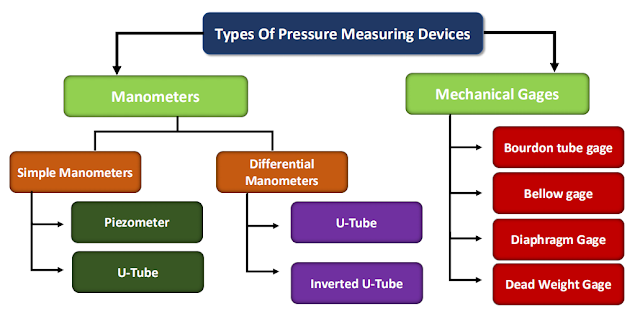Piezometer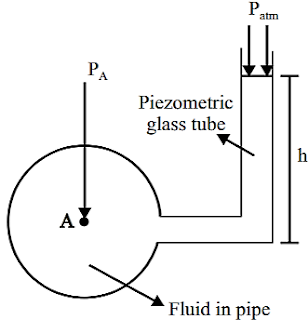➢ Piezometer is a simple manometric device which measures pressure at a point in a fluid, without balancing any other fluid column.

➢ Connect a piezometer glass tube, at the point where pressure is to be measured, such that the other end of the piezometer tube is open to the atmosphere.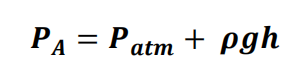## U-tube Simple Manometer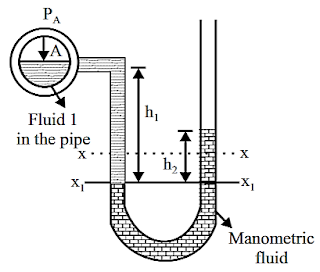➢ U-tube manometer is a simple manometric device used to measure pressure at a point in a fluid, by balancing the fluid column by the same or another column of fluid. U-tube Simple Manometer

➢ It has a glass tube bent in “U” shape with some amount of same or other type of fluid, called manometric fluid like mercury.

➢ Pressure equilibrium at section X1 –X1 :

### Differential U-tube Manometer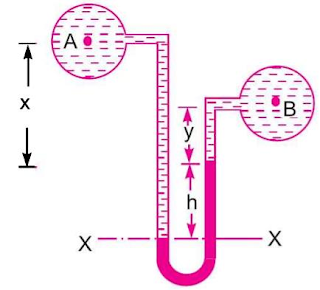➢ It measures pressure difference ∆P at two different points “A” and “B” in a single pipe or different pipes, carrying two fluids of same or different kinds. Differential U-tube Manometer

➢ Pressure equilibrium at section X – X :

### Inverted Differential U-tube Manometer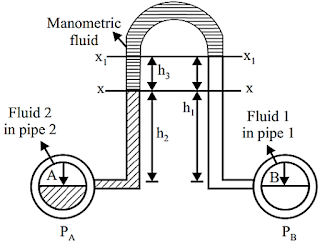➢ Pressure equilibrium at section X1 – X1 :

## Force on Plane Surface

➢ A plate exposed to a liquid, such as a gate valve in a dam, the wall of a liquid storage tank is subjected to fluid pressure distributed over its surface

➢ On a plane surface, the hydrostatic forces form a system of parallel forces, and we often need to determine the magnitude of the force and its point of application, which is called the center of pressure.

➢ The magnitude of the resultant force acting on a plane surface of a completely submerged plate in a homogeneous (constant density) fluid is equal to the product of the pressure at the centroid of the surface and the area A of the surface.

### Center of pressure

➢ Point at which resultant force is acting. It is given by :

## Area moment of Inertia of some important objects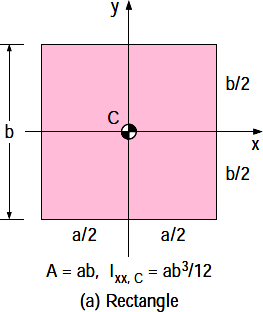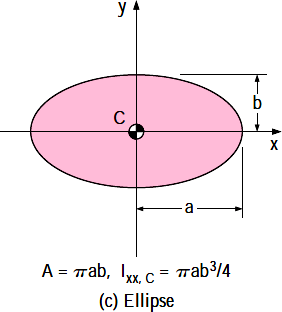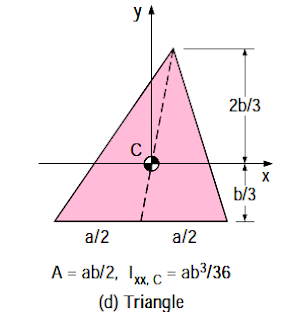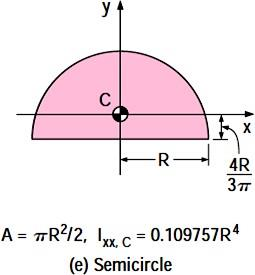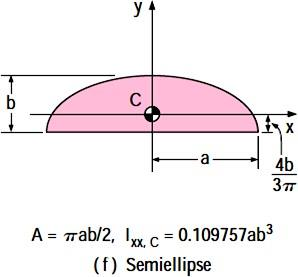## Force on Curved Surface

➢ For a submerged curved surface, the determination of the resultant hydrostatic force is more involved since it typically requires the integration of the pressure forces that change direction along the curved surface.

➢ The easiest way to determine the resultant hydrostatic force FR acting on a two-dimensional curved surface is to determine the horizontal and vertical components FH and FV separately

➢ Force on Curved Surface in y-direction(Vertical force) is given by Weight of liquid supported by the curved surface up to free surface of liquid.

➢ Here V = volume of liquid supported by the curved surface up to free surface of liquid.

➢ Force on Curved Surface in x-direction(horizontal force) is given by Total force on the projected area on the vertical plane.

➢ Here A = projected area on the vertical plane.

ħ = centroid distance from free surface of projected area.

## Buoyancy

➢ The buoyant force acting on a body immersed in a fluid is equal to the weight of the fluid displaced by the body, and it acts upward through the centroid of the displaced volume.

➢ This is known as Archimedes’ principle, after the Greek mathematician Archimedes (287–212 BC),and is expressed as :

➢ Here v̅ = volume of liquid displaced by the body.

ρl= density of the liquid.

➢ If body is in equilibrium :

➢ Here v = volume of the body.

ρ = density of the body.

## Floatation

➢ A body immersed in a fluid :

➢ Sinks to the bottom when its density is greater than the density of the fluid.

➢ Remains at rest at any point in the fluid when its density is equal to the density of the fluid.

➢ Rises to the surface of the fluid and floats when the density of the body is less than the density of the fluid

## Center of Buoyancy

➢ It is the point at which buoyant force is acting.

➢ It is the center of gravity of the volume of liquid displace by the body.

## Stability of Submerged Body

➢ A fully submerged body can be in stable, unstable and neutral equilibrium according to relative position of the center of gravity if the body and center of buoyancy of the body.

➢ A body is said to be in a stable equilibrium position if, when displaced, it returns to its equilibrium position.

➢ Conversely, it is in an unstable equilibrium position if, when displaced (even slightly), it moves to a new equilibrium position.

➢ And it is in a neutral equilibrium position if, when displaced, it does not return to a previous equilibrium position, and stays there.

➢ For the completely submerged body shown, which has a center of gravity below

the center of buoyancy, a rotation from its equilibrium position will create a restoring couple formed by the weight ,and the buoyant force, which causes the body to rotate back to its original position.

➢ Thus, for this configuration the body is stable. It is to be noted that as long as the center of gravity falls below the center of buoyancy, this will always be true; that is, the body is in a stable equilibrium position with respect to small rotations.

➢ If the center of gravity of the completely submerged body is above the center of buoyancy, the resulting couple formed by the weight and the buoyant force will cause the body to overturn and move to a new equilibrium position.

➢ Thus, a completely submerged body with its center of gravity above its center of buoyancy is in an unstable equilibrium position.

➢ If the center of gravity of the completely submerged body and the center of buoyancy is at same point, there will be no resulting couple can be created by disturbing the body.

➢ Thus, a completely submerged body with its center of gravity and the center of buoyancy is at same point, is in an neutral equilibrium position.

## Forces on partially Submerged Bodies

### Meta Centre

➢ It is defined as the point about which a body starts oscillating when the body is tilted by a small angle.

➢ The meta centre may also be defined as the point at which the line of action of the force of buoyancy will meet the normal axis of the body when the body is given a small angular displacement.

### Meta centric height

➢ The distance MG i.e. the distance between the meta centre of a floating body and the centre of gravity of the body is called meta centric height.

GM =BM - BG

➢ BG can be determined by geometry.

➢ BM is given by :

➢ Here I= minimum area moment of inertia.

➢ ̅V= volume displaced by the body

## Stability of Floating Body

➢ The stability of a floating body is determined from the position of Meta centre (M). In case of floating body, the weight of the body is equal to the weight of liquid displaced.

Stable Equilibrium : if the point M is above G, the floating body will be in stable equilibrium.

Unstable Equilibrium – If the point M is below G, the floating body will be in unstable equilibrium.

Neutral Equilibrium – If the point M is at the centre of gravity of the body, the floating body will be in neutral equilibrium

## Fluid Kinematics

➢ Kinematics of fluid describes the fluid motion and its consequences without consideration of the nature of forces causing the motion.

## METHODS OF DESCRIBING FLUID MOTION

➢ The fluid motion is described by two methods.

### Lagrangian Method

➢ In the Lagrangian method, a single fluid particle is followed during its motion.

### Eulerian Method

➢ In Eulerian Method the velocity, acceleration, pressure, density etc., are described at a point in flow field. The Eulerian method is commonly used in fluid mechanics.

## TYPES OF FLUID FLOW

Steady flow is defined as that type of flow in which the fluid characteristics like velocity, pressure,density, etc., at a point do not change with time. Thus for steady flow, mathematically:

Unsteady flow is that type of flow, in which the velocity, pressure or density at a point changes with respect to time.

### UNIFORM AND NON UNIFORM FLOWS

Uniform flow is defined as that type of flow in which the velocity at any given time does not change with respect to space (i.e. length of direction of the flow), Mathematically, for uniform flow

Non uniform flow is that type of flow in which the velocity at any given time changes with respect to space.

### LAMINAR AND TURBULENT FLOWS

Laminar flow is defined as that type of flow in which the fluid particles move along well defined paths or stream line and all the stream lines are straight and parallel. Thus the particles move in

laminas or layers gliding smoothly over the adjacent layer. This type of flow is also called stream line flow or viscous flow

Turbulent flow is that type of flow in which the fluid particles move in a zig-zag way. Due to movement in zig-zag way, the eddies formation takes place which are responsible for high energy Loss

### COMPRESSIBLE AND INCOMPRESSIBLE FLOWS

Compressible flow is that type of flow in which the density of the fluid changes from point to point or in other words the density is not constant for the fluid. Thus, mathematically, for compressible flow

➢ ρ ≠ Constant

Incompressible flow is that type of flow in which the density is constant for the fluid flow. Liquids are generally incompressible while gases are compressible. Mathematically, for incompressible flow

➢ ρ = Constant

### ROTATIONAL AND IRROTATIONAL FLOWS

Rotational flow is that type of flow in which the fluid particles while flowing along stream-lines, also rotate about their own axis.

➢ And if the fluid particles while flowing along streams-lines, do not rotate about their own axis then that type of flow is called irrotatation flow.

### ONE TWO AND THREE DIMENSIONAL FLOWS

➢ One dimensional flow is that type of flow in which the flow in which the flow parameter such as velocity is a function of time and one space coordinate only, say x.

➢ Two dimensional flow is that type of flow in which the flow in which the flow parameter such as velocity is a function of time and two space coordinate only, say x and y.

➢ Two dimensional flow is that type of flow in which the flow in which the flow parameter such as velocity is a function of time and two space coordinate only, say x and y.

➢ Three dimensional flow is that type of flow in which the flow in which the flow parameter such as velocity is a function of time and three space coordinate only, say x , y and z.

## QUESTION FROM THESE TOPICS

1.Liquids transmit pressure equally in all the directions. This is according to
(A) Boyle’s law
(B) Archimedes law
(C) Pascal’s law
(D) Newton’s formula

2.A manometer is used to measure
(A) Velocity of flow in channel
(B) Atmospheric pressure
(C) Pressure in pipes
(D) None of these

3.A pressure of 25 m of water is equal to
(A) 25 kN/m²
(B) 245 kN/m²
(C) 2500 kN/m²
(D) None of these

4.The normal stress is same in all directions at a point in a fluid
(A) Only when the fluid is frictionless
(B) Only when the fluid is incompressible and has zero viscosity
(C) When there is no motion of one fluid layer relative to an adjacent layer
(D) Irrespective of the motion of one fluid layer relative to an adjacent layer

5.The intensity of pressure in a liquid due its depth will, vary with depth
(A) Directly
(B) Indirectly
(C) Both directly and indirectly
(D) None of these

6.The pressure gauges G1 and G2 installed on the system show pressures of PG1 = 5.00 bar and PG2 = 1.00 bar. The value of unknown pressure P is?(Atmospheric pressure 1.01 bars)
(a) 1.01 bar
(b) 2.01 bar
(c) 5.00 bar
(d) 7.01 bar

7.The pressure difference of two very light gasses in two rigid vessels is being measured by a vertical U-tube water filled manometer. The reading is found to be 10 cm. what is the pressure difference?
(a) 9.81 kPa (b) 0.0981 bar (c) 98.1 Pa (d) 981 N/m2

8.The standard atmospheric pressure is 762 mm of Hg. At a specific location, the barometer reads 700 mm of Hg. At this place, what does at absolute pressure of 380 mm of Hg correspond to?
(a) 320 mm of Hg vacuum (b) 382 of Hg vacuum
(c) 62 mm of Hg vacuum (d) 62 mm of Hg gauge

9.In an open U tube containing mercury, kerosene of specific gravity 0·8 is poured into one of its limbs so that the length of column of kerosene is about 40 cm. The level of mercury column in that limb is lowered approximately by how much?
(a) 2·4 cm (b) 1·2 cm (c) 3·6 cm (d) 0·6 cm

10.In given figure, if the pressure of gas in bulb A is 50 cm Hg vacuum and Patm = 76 cm Hg, then height of column H is equal to
(a) 26 cm (b) 50 cm
(c) 76 cm (d) 126 cm

11.A rectangular water tank, full to the brim, has its length, breadth and height in the ratio of 2: 1: 2. The ratio of hydrostatic forces at the bottom to that at any larger vertical surface is:
(a) 1/2 (b) 1 (c) 2 (d) 4

12.A circular plate 1.5 m diameter is submerged in water with its greatest and least depths below the surface being 2 m and 0.75 m respectively. What is the total pressure(approximately) on one face of the plate?
(a) 12kN (b) 16kN (c) 24kN (d) None of the above

13.The vertical component of pressure force on a submerged curved surface is equal to

(A) Weight of liquid vertically above the curved surface and extending upon the free surface
(B) The force on a vertical projection of the curved surface
(C) The product of pressure at centroid and surface area
(D) Horizontal component

14.The depth of centre of pressure for a rectangular lamina immersed vertically in water up to
height ‘h’ is given by:
(a) h/2 (b) h/4
(c)2h/3 (d) 3h/2

15.The hydrostatic force on the curved surface AB shown in given figure acts.
(a) Vertically downwards
(b) Vertically upwards
(c) Downwards, but at an angle with the vertical plane.
(d) Upwards, but at an angle with the vertical plane

16.The resultant upward pressure of a fluid on a floating body is equal to the weight of fluid displaced by the body. This definition is according to ____ .
(A) Buoyancy
(B) Equilibrium of a floating body
(C) Archimedes principle
(D) Bernoulli’s theorem

17.A balloon lifting in air follows the _____ .
(A) Law of gravitation
(B) Archimedes principle
(C) Principle of buoyancy
(D) All options are correct

18.An object weight 60 gm in air 50 gm in water and 40 gm in oil. Then the specific gravity of the oil will be _____ .
(A) 0.25
(B) 1
(C) 1.5
(D) 2

19.A block of ice floating over water in a vessel slowly melts in, the water level in the vessel will
(A) Start rising
(B) Start falling
(C) Will remains constant
(D) Will depend on temperature of water.

20.A floating body is said to be in a state of stable equilibrium.
(A) When its metacentric height is zero
(B) When the metacenter is above the center of gravity
(C) When the metacenter is below the centre of gravity
(D) Only when its centre of gravity is below it centre of buoyancy

21.When a body floating in a liquid is displaced slightly, it oscillates about ____ .
(A) Centre of gravity of body
(B) Centre of pressure
(C) Centre of buoyancy
(D) Meta center

22.A barge 30m long and 10m wide has a draft of 3m when flowing with its sides in vertical position. If its centre of gravity is 2.5m above the bottom, the nearest value of meta centric height is:
(a) 3.28m (b) 2.78m (c) 1.78m (d) Zero

Thank you visit and share..